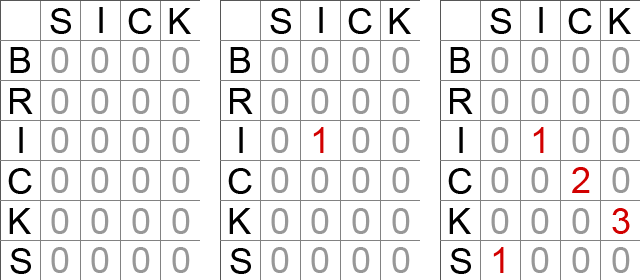# Solving the longest common substring problem with AS3

The longest common substring problem is to find the longest string (or strings) that is a substring (or are substrings) of two strings.

This means if we have two strings, let’s say “ampersand” and “misunderstanding”, the result is given by “ers” and “and”. It may seem a silly exercise but it’s the basic of most text revision software.

Let’s see how to solve it: just like for the Levenshtein distance, we need a matrix where to place both strings.

We’ll make the example with “sick” and “bricks”. Look at the picture:From left, to right, we fill the matrix with zeros, then from row to row starting from the upper to the lower we scan all characters from left to right looking for matches. If letters match, the corresponding value will take 1+the value of its upper left cell. At the end we’ll select the element with the higher number, and in going backward in the diagonal we’ll find the longest common substring. If more cells have the same value, there will be more than one solution to the problem. In this case is “ick”

Let’s see it running with AS3:

```package {
import flash.display.Sprite;
import flash.text.TextField;
import flash.text.TextFieldType;
import flash.text.TextFormat;
import flash.events.Event;
public class longest extends Sprite {
private var from:TextField=new TextField();
private var to:TextField=new TextField();
private var lcs:TextField=new TextField();
private var text_format:TextFormat = new TextFormat();
public function longest() {
text_format.color=0x000000;
text_format.size=24;
with (from) {
type=TextFieldType.INPUT;
x=20;
y=20;
width=460;
height=30;
text="string";
border=true;
setTextFormat(text_format);
}
with (to) {
type=TextFieldType.INPUT;
x=20;
y=70;
width=460;
height=30;
text="substring";
border=true;
setTextFormat(text_format);
}
with (lcs) {
x=20;
y=120;
width=460;
height=30;
text="LCS = "+strlcs(from.text,to.text);
setTextFormat(text_format);
}
}
private function strlcs(from:String,to:String):String {
// finding lengths of both strings
var from_len:uint=from.length;
var to_len:uint=to.length;
// longest is the array which will contain the longest string(s)
var longest:Array=new Array();
// lcs is the matrix used to determine the longest string(s)
var lcs:Array=new Array();
// largest_til_now is the largest match found until now
var largest_til_now:uint=0;
// if one of the strings is empty, then we can't run the function
if (from_len==0||to_len==0) {
return "-";
}
// filling the matrix with zeros
for (var i:uint=0; ilargest_til_now) {
// update the largest match and empty the array
largest_til_now=lcs[i][j];
longest=new Array();
}
// if this element has the same value of the largest match...
if (lcs[i][j]==largest_til_now) {
// add it to matches array
longest.push(from.substr(i-largest_til_now+1,largest_til_now));
}
}
}
}
// return matches array
return longest.toString();
}
public function on_change(e:Event):void {
lcs.text="LCS = "+strlcs(from.text,to.text);
lcs.setTextFormat(text_format);
}
}
}```

The core of the script is `strlcs` function which is fully commented.

This is the result:

Change the strings in the text fields to see how the result updates.

214 GAME PROTOTYPES EXPLAINED WITH SOURCE CODE
// 1+2=3
// 10000000
// 2 Cars
// 2048
// Avoider
// Ballz
// Block it
// Blockage
// Bloons
// Boids
// Bombuzal
// Breakout
// Bricks
// Columns
// CubesOut
// Dots
// DROP'd
// Dudeski
// Eskiv
// Filler
// Fling
// Globe
// HookPod
// Hundreds
// InkTd
// Iromeku
// Lumines
// Magick
// MagOrMin
// Maze
// Memdot
// Nano War
// Nodes
// o:anquan
// Ononmin
// Pacco
// Phyballs
// Platform
// Poker
// Pool
// Poux
// Pudi
// qomp
// Racing
// Renju
// SameGame
// Security
// Sling
// Slingy
// Sokoban
// Splitter
// Sproing
// Stack
// Stringy
// Sudoku
// Tetris
// Threes
// Toony
// Turn
// TwinSpin
// vvvvvv
// Wordle
// Worms
// Yanga
// Zhed
// zNumbers